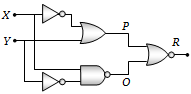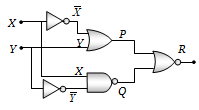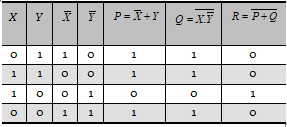Figure gives a system of logic gates. From the study of truth table it can be found that to produce a high output (1) at R, we must have(a) X = 0, Y = 1
(b) X = 1, Y = 1
(c) X = 1, Y = 0
(d) X = 0, Y = 0

(c)The truth table can be written asHence X = 1, Y = 0 gives output R =1

Difficulty Level:

• 11%
• 29%
• 50%
• 11%